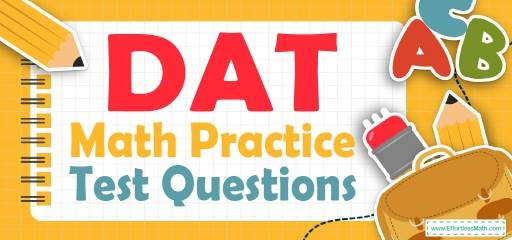# DAT Quantitative Reasoning Math Practice Test QuestionsPreparing for the DAT Quantitative Reasoning Math test? Looking for sample DAT Math questions to help you get ready for the DAT quantitative reasoning test? Try these free DAT Quantitative Reasoning Math Practice questions. Reviewing practice questions is the best way to brush up on your Math skills. Here, we walk you through solving 10 common DAT Quantitative Reasoning Math practice problems covering the most important math concepts on the DAT Quantitative Reasoning Math test.

These DAT Quantitative Reasoning Math practice questions are designed to be similar to those found on the real DAT Quantitative Reasoning Math test. They will assess your level of preparation and will give you a better idea of what to study on your exam.

## 10 Sample DAT Quantitative Reasoning Math Practice Questions

1- If tan $$\theta = \frac{5}{12}$$ and sin $$\theta > 0$$, then cos $$\theta$$ = ?

A. $$-\frac{5}{13}$$

B. $$\frac{12}{13}$$

C. $$\frac{13}{12}$$

D. $$-\frac{12}{13}$$

E. 0

2- If $$60\%$$ of x equal to $$30\%$$ of 20, then what is the value of $$(x+5)^2$$?

A. 25.25

B. 26

C. 26.01

D. 2025

E. 225

3- In the $$xy$$-plane, the point $$(4,3)$$ and $$(3,2)$$ are on line $$A$$. Which of the following equations of lines is parallel to line $$A$$?

A. $$y=3x$$

B. $$y=10$$

C.$$y=\frac{x}{2}$$

D. $$y=2x$$

E. $$y=x$$

4- When point $$A (10, 3)$$ is reflected over the y-axis to get the point $$B$$, what are the coordinates of point $$B$$?

A. $$(10, 3)$$

B. $$(-10, -3)$$

C. $$(-10, 3)$$

D. $$(10, -3)$$

E. $$(0, 3)$$

5- If the area of a trapezoid is 100, what is the perimeter of the trapezoid?

A. 25

B. 35

C. 45

D. 55

E. 65

6- If $$f(x)=2x^3+5x^2+2x$$ and $$g(x)=-2$$, what is the value of $$f(g(x))$$?

☐A. 36

☐B. 32

☐C. 24

☐D. 4

☐E. 0

7- In the following equation when z is divided by 3, what is the effect on $$x$$?
$$x = \frac{8y + \frac{r}{r + 1}}{\frac{6}{z}}$$

A. x is divided by 2

B. x is divided by 3

C. x does not change

D. x is multiplied by 3

E. x is multiplied by 2

8- A boat sails 40 miles south and then 30 miles east. How far is the boat from its start point?

A. 45 miles

B. 50 miles

C. 60 miles

D. 70 miles

E. 80 miles

9- $$x$$ is $$y\%$$ of what number?

A. $$\frac{100x}{y}$$

B. $$\frac{100y}{x}$$

C. $$\frac{x}{100y}$$

D. $$\frac{y}{100x}$$

E. $$\frac{xy}{100}$$

10- If the cotangent of an angle $$β$$ is 1, then the tangent of angle $$β$$ is

A. $$-1$$

B. 0

C. 1

D. 2

E. 3

## Best DAT Quantitative Reasoning Math Prep Resource for 2022

1- B
$$tanθ=\frac{opposite}{adjacent}$$
$$tanθ=\frac{5}{12}$$⇒ we have the following right triangle. Then
$$c=√(5^2+12^2 )=√(25+144)=√169=13$$
$$\frac{adjacent}{hypotenuse}=\frac{12}{13}$$

2- E
$$0.6x=(0.3)×20→x=10→(x+5)^2=(15)^2=225$$

3- E
The slop of line A is:$$m= \frac{y_2-y_1}{x_2-x_1}=\frac{3-2}{4-3}=1$$
Parallel lines have the same slope and only choice $$E (y=x)$$ has slope of 1.

4- C
When points are reflected over $$y$$-axis, the value of $$y$$ in the coordinates doesn’t change and the sign of $$x$$ changes. Therefore, the coordinates of point $$B$$ is $$(-10,3)$$.

5- B
The area of trapezoid is:
$$(\frac{8+12}{2})×x=100→10x=100→x=10$$
$$y=√(3^2+4^2 )=5$$
$$Perimeter \space is: 12+10+8+5=35$$

6- E
$$g(x)=-2, then f(g(x))= f(-2)=2 (-2)^3+5(-2)^2+2(-2)= -16+20-4=0$$

7- B
$$x = \frac{8y + \frac{r}{r + 1}}{\frac{6}{\frac{z}{3}}}$$
$$x = \frac{8y + \frac{r}{r + 1}}{\frac{3×6}{z}}$$
$$\frac{1}{3}×\frac{8y +\frac{r}{r + 1}}{\frac{6}{z}}=\frac{x}{3}$$

8- B
Use the information provided in the question to draw the shape.
Use Pythagorean Theorem: $$a^2 + b^2 = c^2$$
$$402 + 302 = c2 ⇒ 1600 + 900 = c2 ⇒ 2500 = c2 ⇒ c = 50$$

9- A
Let the number be $$A$$. Then:
$$x=y\% ×A$$
Solve for $$A$$.
$$x = \frac{y}{100}×A$$
Multiply both sides by $$100/y$$:
$$x×\frac{100}{y}=\frac{y}{100}×\frac{100}{y}×A$$
$$A=\frac{100x}{y}$$

10- C
$$tangent β=\frac{1}{cotangent β}=1$$

Looking for the best resource to help you succeed on the DAT Quantitative Reasoning Math test?

## The Best Books to Ace the DAT Quantitative Reasoning Math Test

### What people say about "DAT Quantitative Reasoning Math Practice Test Questions - Effortless Math: We Help Students Learn to LOVE Mathematics"?

No one replied yet.

X
23% OFF

Limited time only!

Save Over 23%

SAVE $5 It was$21.99 now it is \$16.99